# GSEB Solutions Class 8 Maths Chapter 16 Playing with Numbers Intex Questions

Gujarat Board GSEB Textbook Solutions Class 8 Maths Chapter 16 Playing with Numbers Intex Questions and Answers.

## Gujarat Board Textbook Solutions Class 8 Maths Chapter 16 Playing with Numbers Intex Questions

Try These (Page 250)

Question 1.
Write the following numbers in generalised form?

1. 25
2. 73
3. 129
4. 302

Solution:
1. 25 = 20 + 5 = 10 × 2 + 5 × 1
= 10 × 2 + 5

2. 73 = 70 + 3
= 10 × 7 + 3 × 1
= 10 × 7 + 3

3. 129 = 100 + 20 + 9
= 100 × 1 + 10 × 2 + 1 × 9
= 100 × 1 + 10 × 2 + 9

4. 302 = 100 × 3 + 10 × 0 + 1 × 2 = 300 + 0 + 2Question 2.
Write the following in the usual form?

1. 10 × 5 + 6
2. 100 × 7 + 10 × 1 + 8
3. 100 × a + 10 × c + b

Solution:

1. 10 × 5 + 6 = 50 + 6 = 56
2. 100 × 7 + 10 × 1 + 8 = 700 + 10 + 8 = 718
3. 100 × a + 10 × c + b = 100a + 10c + b
=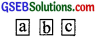Try These (Page 251)
[Adding the number with reversed digits to the chosen numbers.]

Question 1.
Check what the result would have been if Sundaram had chosen the numbers shown below?

1. 27
2. 39
3. 64
4. 17

Solution:
1. Chosen number = 27
Number with reversed digits = 72
Sum of the two numbers = 27 + 72 = 99
Now, 99 = 11  = 11 [2 + 7]
= 11 [Sum of the digits of the chosen number]

2. Chosen number = 39
Number with reversed digits = 93
Sum of the two numbers = 39 + 93 = 132
Now, 132 + 11 = 12
i. e., 132 = 11 = 11 [3 + 9]
= 11 [Sum of the digits of the chosen number]

3. Chosen number = 64
Number with reversed digits = 46
Sum = 64 + 46 = 110
Now, 110 = 11 = 11 [6 + 4]
= 11 [Sum of the digits of the chosen number]

4. Chosen number = 17
Number with reversed digits = 71
Sum = 17 + 71 = 88
Now, 88 = 11 = 11[1 + 7]
= 11 [Sum of the digits of the chosen number]

Try These (Page 251)
[Finding the difference of the chosen number and the number obtained by reversing the digits.]

Question 1.
Check what result would have been if Sundaram had chosen the numbers shown?

1. 17
2. 21
3. 96
4. 37

Solution:
1. Chosen number =17
Number with reversed digits = 71
Difference = 71 – 17 = 54 = 9 × 
= 9 × [Difference of the digits of the chosen number (7 – 1 = 6)]

2. Chosen number = 21
Number with reversed digits = 12
Difference = 21 – 12 = 9 = 9 × 
= 9 × [Difference between the digits of the chosen number (2 – 1 = 1)]

3. Chosen number = 96
Number with reversed digits = 69
Difference = 96 – 69 = 27 = 9 × 
= 9 × [Difference between the digits of the chosen number (9 – 6 = 3)]

4. Chosen number = 37
Number with reversed digits = 73
Difference = 73 – 37 = 36 = 9 × 
= 9 × [Difference between the digits of the chosen number (7 – 3 = 4)]Try These (Page 252)

Question 1.
Check what the result would have been if Minakshi had chosen the numbers shown below. In each case keep a record of the quotient obtained at the end?

1. 132
2. 469
3. 737
4. 901

Solution:
1. 132 Chosen number = 132
Reversed number = 231
Difference = 231 – 132 = 99
We have 99 ÷ 99 = 1, remainder = 0

2. 469 Chosen number = 469
Reversed number = 964
Difference = 964 – 469 = 495
We have 495 ÷ 99 = 5, remainder = 0

3. Chosen number = 737
Reversed number = 737
We have Difference = 737 – 737 = 0
0 ÷ 99 = 0, remainder = 0

4. Chosen number = 901
Reversed number = 109
Difference = 901 – 109 = 792
We have 792 ÷ 99 = 8, remainder = 0
Forming three-digit number with given three digits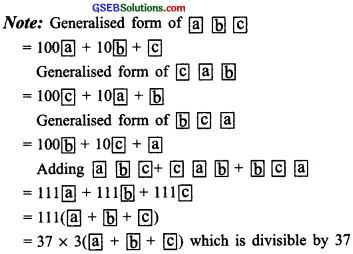Try These (Page 253)

Question 1.
Check what the result would have been if Sundaram had chosen the numbers shown below?

1. 417
2. 632
3. 117
4. 937

Solution:
1. Chosen number = 417
Two other numbers with the same digits are 741 and 174
Sum of the three numbers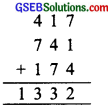We have 1332 ÷ 37 = 36, remainder

2. Chosen number = 632
Two other numbers are 263 and 326
Sum of the three numbers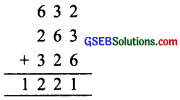We have 1221 ÷ 37 = 33, remainder = 0

3. Chosen number =117
Other numbers are 711 and 171
Sum of the three numbers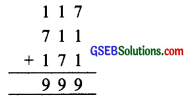We have 999 ÷ 37 = 27, remainder = 0

4. Chosen number = 937
Other two numbers are 793 and 379
∴ Sum of the three numbers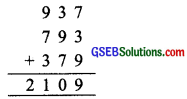We have 2109 ÷ 37 = 57, remainder = 0

Try These (Page 257)

Question 1.
If the division N ÷ 5 leaves a remainder of 1, what might be the ones digit of N?
Solution:
The ones digit, when divided by 5, must leave a remainder of 3. So the ones digit must be either 3 or 8.

Question 2.
If the division N ÷ 5 leaves a remainder of 1, what might be the ones digit of N?
Solution:
If remainder = 1, then the ones digit of ‘N’ must be either 1 or 6.

Question 3.
If the division N ÷ 5 leaves a remainder of 4, what might be the ones digit of N?
Solution:
If remainder = 4, then the ones digit of ‘N’ must be either 9 or 4.

Try These (Page 257)

Question 1.
If the division N ÷ 2 leaves a remainder of 1, what might be the ones digit of N?
Solution:
N is odd; so its ones digit is odd. Therefore. the ones digit must be 1, 3, 5, 7 or 9.

Question 2.
If the division N ÷ 2 leaves no remainder (i.e.. zero remainder), what might be the one digit of N?
Solution:
∵ Remainder = 0
∴ Ones digit can be 0, 2, 4, 6 or 8.Question 3.
Suppose that the division N ÷ 5 leaves a remainder of 4 and the division N ÷ 2 leaves a remainder of I. What must be the ones digit of N?
Solution:
∵ N + 5 and remainder = 4
∴ Ones digit can be 4 or 9.
Again N ÷ 2 and remainder = 1
∴ N must be an odd number.
Thus, ones digit can be 9 only.

Try These (Page 259)

Question 1.
Check the divisibility of the following numbers by 9?
1. 108
2. 616
3. 294
4. 432
5. 927
Solution:
1. 108
∵ 1 + 0 + 8 = 9
and 9 is divisible by 9.
[∵ 9 + 9 = 1 and remainder = 0]
∴ 108 is divisible by 9.

2. 616
We have 6 + 1 + 6 = 13
and 13 ÷ 9 = 1, remainder = 4
i.e., 13 is not divisible 9.
∴ 616 is also not divisible by 9.

3. 294
We have 2 + 9 + 4 = 15
and 15 + 9 = 1, remainder = 6
i.e., 15 is not divisible by 9.
∴ 294 is also not divisible by 9.

4. 432
We have 4 + 3 + 2 = 9
9 + 9 = 1, remainder = 0
∴ 432 is divisible by 9.

5. 927
We have 9 + 2 + 7 = 18
and 18 + 9 = 2, remainder = 0
i.e., 18 is divisible by 9.
∴ 927 is also divisible by 9.Try These (Page 260)

Question 1.
Check the divisibility of the following numbers by 3?
1. 108
2. 616
3. 294
4. 432
5. 927
Solution:
1. 108
We have 1 + 0 + 8 = 9
and 9 + 3 = 3, remainder = 0
∴ 108 is divisible by 3.
[∵ 9 is divisible by 3]

2. 616
We have 6 + 1 + 6 = 13
and 13 ÷ 3 = 4, remainder = 1
∴ 13 is not divisible by 3.
Thus 616 is also not divisible by 3.

3. 294
We have 2 + 9 + 4 = 15
and 15 ÷ 3 = 5, remainder = 0
∴ 15 is divisible by 3.
Thus, 294 is also divisible by 3.

4. 432
We have 4 + 3 + 2 = 9
and 9 ÷ 3 = 3, remainder = 0
i.e; 9 is divisible by 3.
Thus, 294 is also divisible by 3.

5. 927
we have 9 + 2 + 7 = 18
and 18 ÷ 3 = 6, remainder = 0
i.e; 18 is divisible by 3.
Thus, 927 is also divisible by 3.